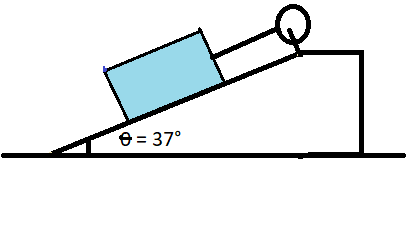# A block is connected to a wheel as shown below. The moment of inertia of the wheel 8.0 kgm^2, and...

## Question:

A block is connected to a wheel as shown below. The moment of inertia of the wheel 8.0 kgm{eq}^2 {/eq}, and its radius is 40 cm. Find the angular acceleration of the wheel caused by downward motion of the 10 kg mass if the frictional force between the mass and the incline is 30 N. Assume that the string wound around the wheel is massless, the wheel turns without friction and the string unwinds without slipping.## Rotational and Linear Motion:

In a complex system, where both linear and rotational motions are present, formulas for linear motion can be translated into formulas for rotational motion by replacing the force with the torque, mass with the moment of inertia, and linear acceleration with the angular acceleration.

## Answer and Explanation:

The equations of motion are given by the following expressions:

{eq}\begin{align*} & m g sin \theta - F_f - T = ma &\text (1) \\ & I \alpha = T R &\text (2) \end{align*} {/eq}

Here

• {eq}m = 10 \ kg {/eq} is the mass of the block;
• {eq}T {/eq} is the tension in the string;
• {eq}\alpha = \dfrac {a}{R} {/eq} is the angular acceleration of the wheel;
• {eq}a {/eq} is the linear acceleration of the block;
• {eq}R = 0.4 \ m {/eq} is the radius of the block;
• {eq}F_f = 30 \ N {/eq} is the friction force between the block and the incline;
• {eq}I = 80 \ kg\cdot m^2 {/eq} is the moment of inertia of the wheel;

Solving for the angular acceleration of the wheel, we obtain:

{eq}\alpha = \dfrac {(mgsin \theta - F_f)R}{I + mR^2} {/eq}

Calculation gives:

{eq}\alpha = \dfrac {(10 \ kg \cdot 9.81 \ m/s^2 \cdot sin (37^{\circ}) - 30 \ N) \cdot 0.4 \ m}{80 \ kg\cdot m^2 + 10 \ kg \cdot (0.4 \ m)^2} \approx 0.14 \ rad/s^2 {/eq}

#### Learn more about this topic:Practice Applying Rotational Motion Formulas

from Physics 101: Help and Review

Chapter 17 / Lesson 15
870